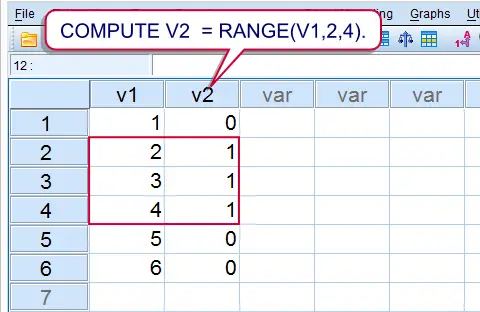SPSS TUTORIALS BASICS ANOVA REGRESSION FACTOR CORRELATION

# SPSS RANGE Function – Quick Tutorial

COMPUTE v2 = RANGE(V1,2,4).SPSS RANGE Function Result

## Summary

SPSS`RANGE` function is used to evaluate whether or not values are within a given range. Test values equal to the lower or upper boundary are also within the given range. Run the syntax below for a quick demonstration.

## SPSS Range Syntax Example

*1. Create couple of cases.

data list free/v1(f1).
begin data
1 2 3 4 5 6
end data.

*2. Check whether value on v2 is between 2 and 4.

compute v2 = range(v1,2,4).
exe.

## Notes

`RANGE` takes three arguments. So in `RANGE(A,B,C)`

• `A` refers to the test value;
• `B` refers to the lower boundary;
• `C` refers to the upper boundary;
• `A`, `B` and `C` can all be values within variables or constants (over cases). The most common scenario, however, is that `A` is a variable and `B` and `C` constants.

`RANGE` may return three values:

• 1 (or “True”) if the test value is within the range;
• 0 (or “False”) if the test value is not within the range;
• A system missing value if the range can't be evaluated due to missing values.

## SPSS Range with Dates and Times

SPSS `RANGE` can be readily used with date variables and time variables. It should be kept in mind that SPSS dates and times are expressed in numbers of seconds. This implies that you should convert “normal” date and time values into numbers of seconds too. This can be done with the `DATE.DMY` and `TIME.HMS` functions as shown in the syntax below.Minutes and seconds default to zero in `TIME.HMS`. That is, `TIME.HMS(18,0,0)` may be shortened to `TIME.HMS(18)`.

## SPSS Range Syntax Example

*1. Create arrival time dataset.

data list free/arrival(time10).
begin data
10:32:12 12:59:43 16:34:36 17:20:50 18:41:23 23:48:03
end data.

*2. Flag arrivals between noon and 6 PM.

compute arrival_during_afternoon = range(arrival,time.hms(12,0,0),time.hms(18)).
exe.

## SPSS Range with Strings

Technically, you can use `RANGE` for string values too. SPSS basically uses an alphabetical order to determine whether string values are in a given range or not. This can be seen by running `SORT CASES` as in the syntax example below.

## SPSS Range Syntax Example

*1. Create mini dataset.

data list free/v1(a2).
begin data
a b c C cc d D EE e f
end data.

*2. Sort cases.

sort cases by v1.

*3. Flag values between 'C' and 'e'.

compute v2 = range(v1,'C','e').
exe.

# Tell us what you think!

*Required field. Your comment will show up after approval from a moderator.

# THIS TUTORIAL HAS 5 COMMENTS:

•### By ratha on October 28th, 2014

Dear Ruben,

Thank you for your encouraging simple tutorials, just joined with the search of RANGE function!

I am working with the counselling client service dataset.

I want to filter records of clients who had visit date for any services within a quarter. The dataset has many variables for dates for example counselling_session_1.......8
befriending_session_1....8
follow_up_session_1....5

Is it possible with RANGE syntax

Thanks,
ratha

•### By Ruben Geert van den Berg on October 28th, 2014

Yes and no. If I understand correctly, you can use RANGE for evaluating whether one date variable has a value within a date range such as a quarter.

If you want to check whether at least one of several date variables has a value within some date range, you could try and use the RANGE function within a LOOP or DO REPEAT over the date variables.

I wrote a tiny example for how to do this, you'll find it here.

HTH!

•### By ratha on October 28th, 2014

Dear Ruben,

Very very thank you for your quick and excellent guide, it was very easy to follow and I have did it. It is my exiting moment to see your quicker and very simple guidance with right example and syntax.

Thank you so much Ruben!

•### By Saimul Islam on December 10th, 2014

how can i calculate range for this situationsi.e
x= 1,2,3,4,5,6,7,8,9,10,11,12..........2000000........
RANGE(x,2,5) is ok
but i want to calculate if x>=700000 using range

•### By Ruben Geert van den Berg on December 10th, 2014

Note that `RANGE(x,y,z)` is basically a shorthand for `x GE y AND x LE z`. That is, it combines two conditions. If you have only a single condition, have a look at IF or perhaps this dichotomizing syntax.